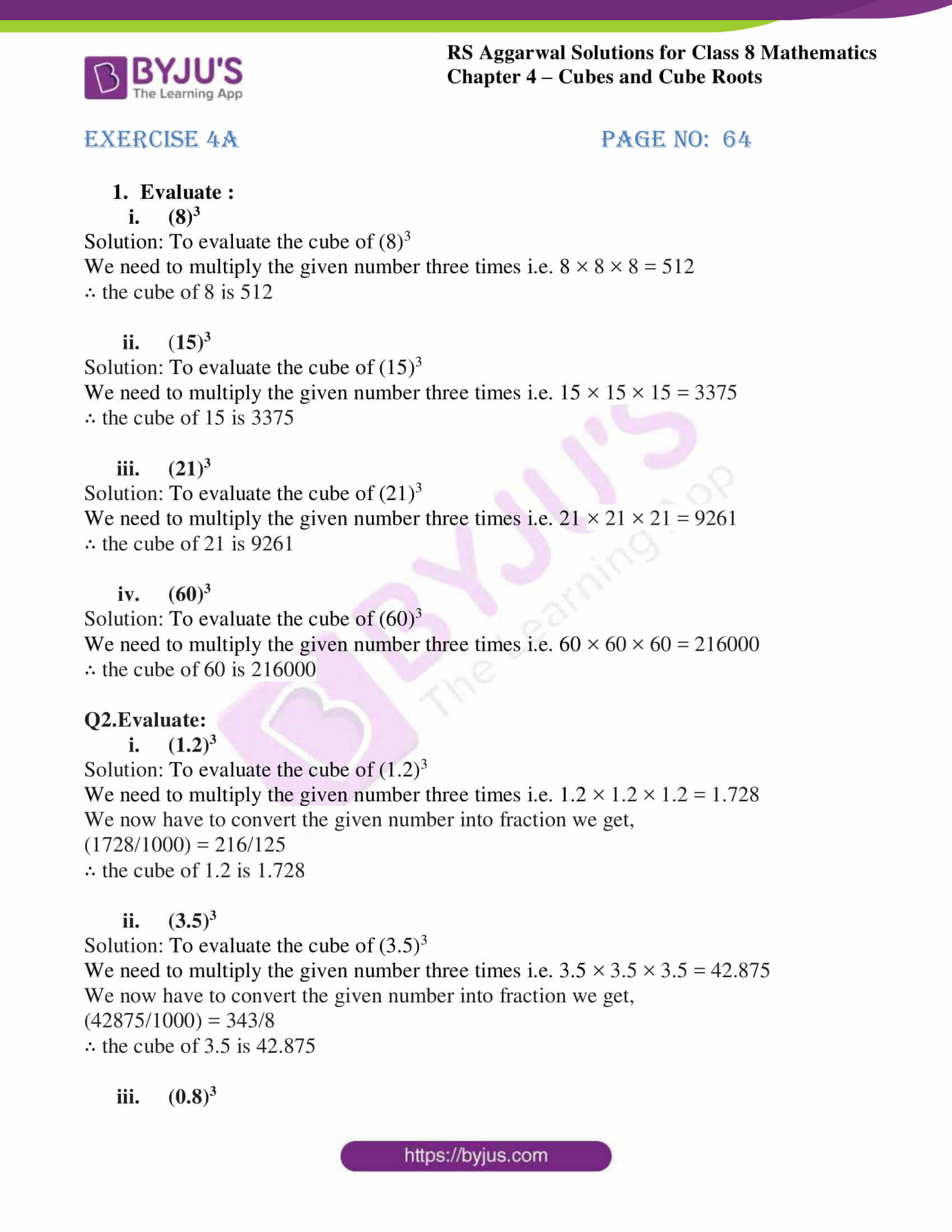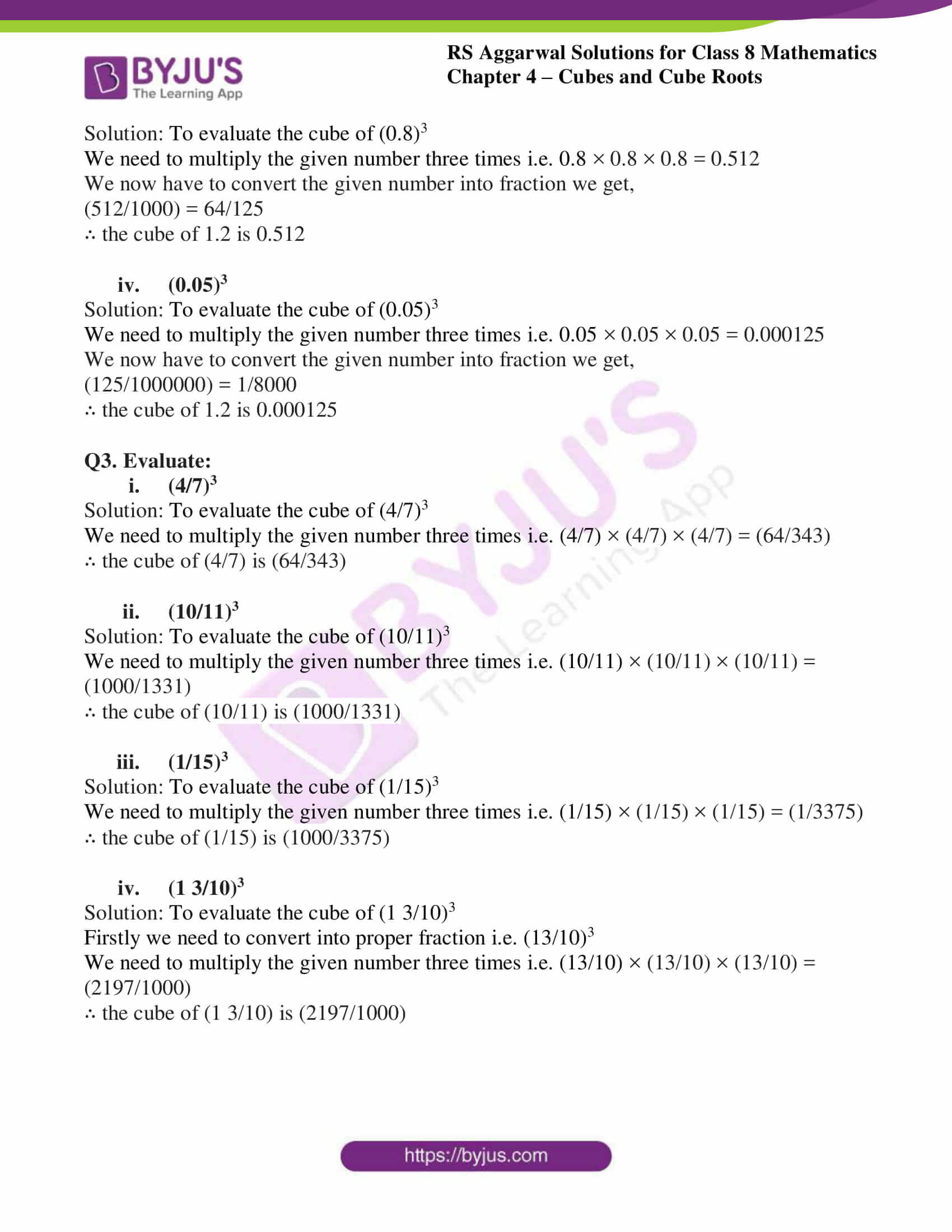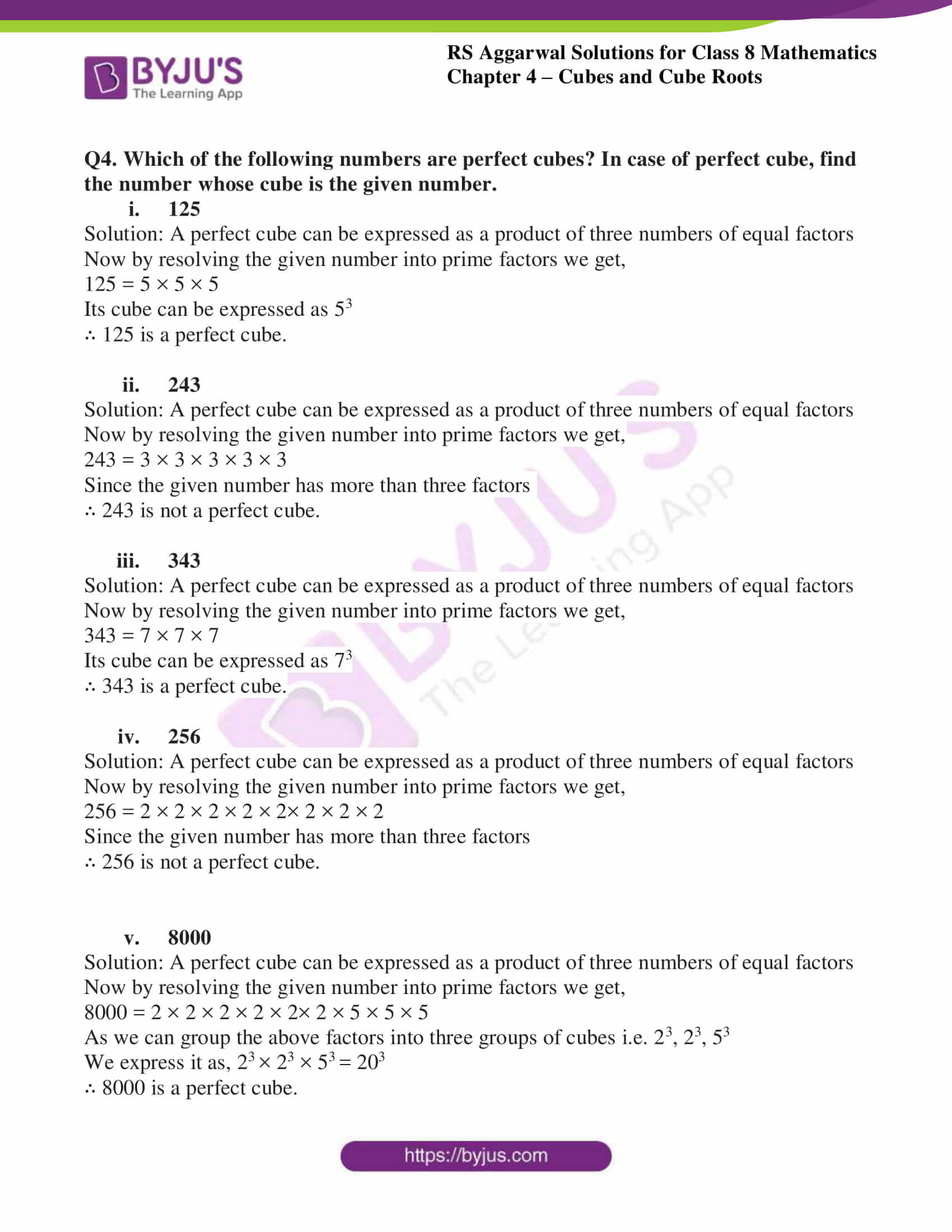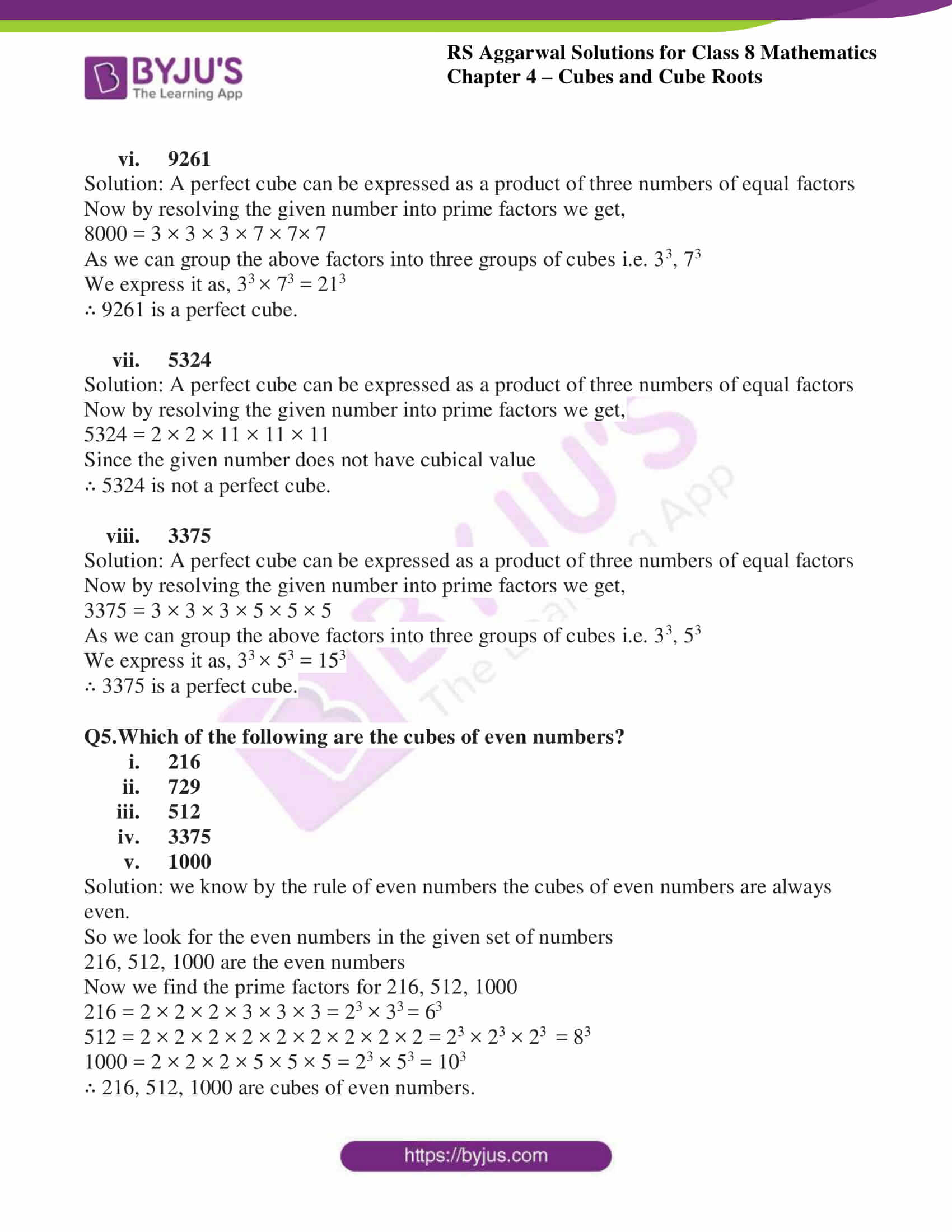# RS Aggarwal Solutions for Class 8 Maths Chapter 4 - Cubes and Cube Roots Exercise 4A

Students can refer and download RS Aggarwal Solutions for Class 8 Maths Chapter 4- Exercise 4A, Cubes and Cube Roots from the links provided below. Our experts have solved the RS Aggarwal Solutions, with the help of which students can practice a large number and different types of questions from the textbook. This would ensure they secure high marks in the examination.

In Exercise 4A of RS Aggarwal Class 8 Maths, we shall study the cube of a number, cubes of negative integers and properties of cubes of numbers. We define a perfect cube as- A given natural number is a perfect cube if it can be expressed as the product of triplets of equal factors.

## Download PDF of RS Aggarwal Solutions for Class 8 Maths Chapter 4- Cubes and Cube Roots Exercise 4A### Access Answers to RS Aggarwal Solutions for Class 8 Maths Chapter 4- Cubes and Cube Roots Exercise 4A

1.Evaluate :

i. (8)3

Solution: To evaluate the cube of (8)3

We need to multiply the given number three times i.e. 8 × 8 × 8 = 512

∴ the cube of 8 is 512

ii. (15)3

Solution: To evaluate the cube of (15)3

We need to multiply the given number three times i.e. 15 × 15 × 15 = 3375

∴ the cube of 15 is 3375

iii. (21)3

Solution: To evaluate the cube of (21)3

We need to multiply the given number three times i.e. 21 × 21 × 21 = 9261

∴ the cube of 21 is 9261

iv. (60)3

Solution: To evaluate the cube of (60)3

We need to multiply the given number three times i.e. 60 × 60 × 60 = 216000

∴ the cube of 60 is 216000

2. Evaluate:

i. (1.2)3

Solution: To evaluate the cube of (1.2)3

We need to multiply the given number three times i.e. 1.2 × 1.2 × 1.2 = 1.728

We now have to convert the given number into fraction we get,

(1728/1000) = 216/125

∴ the cube of 1.2 is 1.728

ii. (3.5)3

Solution: To evaluate the cube of (3.5)3

We need to multiply the given number three times i.e. 3.5 × 3.5 × 3.5 = 42.875

We now have to convert the given number into fraction we get,

(42875/1000) = 343/8

∴ the cube of 3.5 is 42.875

iii. (0.8)3

Solution: To evaluate the cube of (0.8)3

We need to multiply the given number three times i.e. 0.8 × 0.8 × 0.8 = 0.512

We now have to convert the given number into fraction we get,

(512/1000) = 64/125

∴ the cube of 1.2 is 0.512

iv. (0.05)3

Solution: To evaluate the cube of (0.05)3

We need to multiply the given number three times i.e. 0.05 × 0.05 × 0.05 = 0.000125

We now have to convert the given number into fraction we get,

(125/1000000) = 1/8000

∴ the cube of 1.2 is 0.000125

Q3. Evaluate:

i. (4/7)3

Solution: To evaluate the cube of (4/7)3

We need to multiply the given number three times i.e. (4/7) × (4/7) × (4/7) = (64/343)

∴ the cube of (4/7) is (64/343)

ii. (10/11)3

Solution: To evaluate the cube of (10/11)3

We need to multiply the given number three times i.e. (10/11) × (10/11) × (10/11) = (1000/1331)

∴ the cube of (10/11) is (1000/1331)

iii. (1/15)3

Solution: To evaluate the cube of (1/15)3

We need to multiply the given number three times i.e. (1/15) × (1/15) × (1/15) = (1/3375)

∴ the cube of (1/15) is (1000/3375)

iv. (1 3/10)3

Solution: To evaluate the cube of (1 3/10)3

Firstly we need to convert into proper fraction i.e. (13/10)3

We need to multiply the given number three times i.e. (13/10) × (13/10) × (13/10) = (2197/1000)

∴ the cube of (1 3/10) is (2197/1000)

Q4. Which of the following numbers are perfect cubes? In case of perfect cube, find the number whose cube is the given number.

i. 125

Solution: A perfect cube can be expressed as a product of three numbers of equal factors

Now by resolving the given number into prime factors we get,

125 = 5 × 5 × 5

Its cube can be expressed as 53

∴ 125 is a perfect cube.

ii. 243

Solution: A perfect cube can be expressed as a product of three numbers of equal factors

Now by resolving the given number into prime factors we get,

243 = 3 × 3 × 3 × 3 × 3

Since the given number has more than three factors

∴ 243 is not a perfect cube.

iii. 343

Solution: A perfect cube can be expressed as a product of three numbers of equal factors

Now by resolving the given number into prime factors we get,

343 = 7 × 7 × 7

Its cube can be expressed as 73

∴ 343 is a perfect cube.

iv. 256

Solution: A perfect cube can be expressed as a product of three numbers of equal factors

Now by resolving the given number into prime factors we get,

256 = 2 × 2 × 2 × 2 × 2× 2 × 2 × 2

Since the given number has more than three factors

∴ 256 is not a perfect cube.

v. 8000

Solution: A perfect cube can be expressed as a product of three numbers of equal factors

Now by resolving the given number into prime factors we get,

8000 = 2 × 2 × 2 × 2 × 2× 2 × 5 × 5 × 5

As we can group the above factors into three groups of cubes i.e. 23, 23, 53

We express it as, 23 × 23 × 53 = 203

∴ 8000 is a perfect cube.

vi. 9261

Solution: A perfect cube can be expressed as a product of three numbers of equal factors

Now by resolving the given number into prime factors we get,

8000 = 3 × 3 × 3 × 7 × 7× 7

As we can group the above factors into three groups of cubes i.e. 33, 73

We express it as, 33 × 73 = 213

∴ 9261 is a perfect cube.

vii. 5324

Solution: A perfect cube can be expressed as a product of three numbers of equal factors

Now by resolving the given number into prime factors we get,

5324 = 2 × 2 × 11 × 11 × 11

Since the given number does not have cubical value

∴ 5324 is not a perfect cube.

viii. 3375

Solution: A perfect cube can be expressed as a product of three numbers of equal factors

Now by resolving the given number into prime factors we get,

3375 = 3 × 3 × 3 × 5 × 5 × 5

As we can group the above factors into three groups of cubes i.e. 33, 53

We express it as, 33 × 53 = 153

∴ 3375 is a perfect cube.

Q5.Which of the following are the cubes of even numbers?

i. 216
ii. 729
iii. 512
iv. 3375
v. 1000

Solution: we know by the rule of even numbers the cubes of even numbers are always even.

So we look for the even numbers in the given set of numbers

216, 512, 1000 are the even numbers

Now we find the prime factors for 216, 512, 1000

216 = 2 × 2 × 2 × 3 × 3 × 3 = 23 × 33 = 63

512 = 2 × 2 × 2 × 2 × 2 × 2 × 2 × 2 × 2 = 23 × 23 × 23 = 83

1000 = 2 × 2 × 2 × 5 × 5 × 5 = 23 × 53 = 103

∴ 216, 512, 1000 are cubes of even numbers.

### Access other Exercises of RS Aggarwal Solutions for Class 8 Maths Chapter 4- Cubes and Cube Roots

Exercise 4B Solutions 4 Questions

Exercise 4C Solutions 18 Questions

Exercise 4D Solutions 10 Questions

## RS Aggarwal Solutions for Class 8 Maths Chapter 4- Cubes and Cube Roots Exercise 4A

Exercise 4A of RS Aggarwal Class 8, Cubes and Cube Roots contains the basic concepts related to Cubes. This exercise mainly deals with the basic properties of cubes of a number. Some of them include

• The cube of every even number is even.
• The cube of every odd number is odd.
• The cube of a negative integer is always negative.

The RS Aggarwal Solutions can help the students practice diligently while learning the fundamentals as it provides all the answers to the questions from the RS Aggarwal textbook. Students should practice RS Aggarwal Solutions without fail to understand how the concepts are solved. Practicing as many times as possible helps in enhancing time management skills and also boosts the confidence level to achieve high marks.